• 对于位置参数描述正确的是
千次阅读
2018-04-11 00:15:31

1.shell中变量的定义和使用

你可在任何编程语言中使用变量，但是在脚本编程(shell)中它们是没有类型的简称弱类型编程语言，在这个变量中可以保含一个数字，一个字符串，一个单词等。你并不需要声明这个变量，它会在引用这个变量时创建它。

Linux Shell中的变量分为“系统变量”和“用户自定义变量”，可以通过set命令查看系统变量。用$输出变量 老生常谈，变量名命名方法:第一个字符为字母,其余字符可以是字母,数字或下划线，编程都一样。 变量定义:不需要事先定义,直接赋值定义新变量,直接赋值修改原变量的值 0.变量引用:在变量名前加$号,代表变量内容
PATHTOMCAT=/usr/local/apache-tomcat-5.5.23  #上来就是变量名 ，赋值。
echo "path of tomcat is $PATHTOMCAT #这里使用echo命令，回显。用$获取变量值
执行结果： path of tomcat is /usr/local/apache-tomcat-5.5.23
注:
1.定义变量时等号两侧不允许有多余的空格，如果有空格会报错。
[robot@hadoop103 ~]$path1= 'abc' #有空格，报错 bash: abc: command not found... [robot@hadoop103 ~]$ path1='abc'
[robot@hadoop103 ~]$echo$path1
abc

2.定义变量时等号右侧的字符串中含有空格或者制表符,换行符时,要用引号将打算赋值的字符串括起 ，双引号内允许变量替换，而单引号内就不可以
即shell定义字符串变量时字符串可以用单引号，也可以用双引号，也可以不用引号

单引号和双引号的区别

str=yalong
echo "The str is $str" >> The str is yalong #双引号中的$str被其值yalong替换，即双引号允许变量替换
echo 'The str is $str' >> The str is$str #单引号中的内容被原样输出，即单引号不允许变量替换。
转义字符\
echo  "hello world" >>  hello world  #这里面双引号就是双引号，不输出
echo  \"hello world\" >> "hello world"  #输出双引号，这里用了转义字符\所以双引号失去了引号功能，成了字符串。

3.将linux命令返回值赋给变量（重要常用）

[robot@hadoop103 ~]$echo aa=date +%H #这里用的是反转义字符，esc下面的那个符号。 aa=23 [robot@hadoop103 ~]$ echo aa="date+%H" #这里用的是双引号，命令失效。

aa=date+%H

A=ls -la 反引号，运行里面的命令，并把结果返回给变量A
A=$(ls -la) 小括号的功能等价于反引号 A=pwd & echo$A
A=$(pwd)$ echo $A 4.变量使用的常见误区错误 有时候变量名很容易与其他文字混淆，比如： num=2 echo "this is the$numnd"这并不会打印出"this is the 2nd"，而仅仅打印"this is the "，
因为shell会去搜索变量numnd的值，但是这个变量时没有值的。可以使用花括号来告诉shell我们要打印的是num变量： num=2 echo "this is the ${num}nd" 这将打印： this is the 2nd ··  注意这用的是{}来确定变量，不是(),用小括号不行，只能用花括号。括号的的功能等同于反引号 5.变量的设置和查询 A=8 A=abc 定义变量A,可以多次定义，顺序执行 unset A 撤销变量A，unset用来删除变量,unset 命令不能删除只读变量。 变量被删除后不能再次使用。 实例 #!/bin/sh myUrl="http://www.google.com" unset myUrl echo$myUrl
以上实例执行将没有任何输出。
使用 readonly 命令可以将变量定义为只读变量，只读变量的值不能被改变。
下面的例子尝试更改只读变量，结果报错：
#!/bin/bash
myUrl="http://www.baidu.com"
运行脚本，结果出错如下：
/bin/sh: NAME: This variable is read only.
export 变量名  可把变量提升为全局环境变量，可供其他shell程序使用，同样用echo$变量名，查询变量值 这也是环境变量要加export的原因，这样所有的用户都可以使用这个变量 export JAVA_HOME=/usr/local/jdk-7.01 6.位置参数变量和特殊变量(重要） 1.位置参数变量基本语法$n    （功能描述：n为数字，$0代表命令本身，$1-$9代表第一到第九个参数，十以上的参数，十以上的参数需要用大括号包含，如${10}）
$* （功能描述：这个变量代表命令行中所有的参数，$*把所有的参数看成一个整体）
$@ （功能描述：这个变量也代表命令行中所有的参数，不过$@把每个参数区分对待）
$# （功能描述：这个变量代表命令行中所有参数的个数） 2）案例计算输入的参数1和参数2的两个数的和，并输出到控制台 vi aaa.txt，脚本内容如下，然后执行脚本sh aaa.sh 1 2 #!/bin/bash num1=$1
num2=$2 sum=$(( $num1 +$num2))
#变量sum的和是num1加num2
echo $sum #打印变量sum的值，执行脚本，输出的结果就是3. 2）打印输入的参数总数、所有参数 #!/bin/bash echo "A total of$# parameters"
#使用$#代表所有参数的个数 echo "The parameters is:$*"
#使用$*代表所有的参数 echo "The parameters is:$@"
#使用$@也代表所有参数 3）$*与$@的区别 #!/bin/bash for i in "$*"
#$*中的所有参数看成是一个整体，所以这个for循环只会循环一次 do echo "The parameters is:$i"
done
x=1
for y in "$@" #$@中的每个参数都看成是独立的，所以“$@”中有几个参数，就会循环几次 do echo "The parameter$x is: $y" x=$(( $x +1 )) done a）$*和$@都表示传递给函数或脚本的所有参数，不被双引号“”包含时，都以$1 $2 …$n的形式输出所有参数
b）当它们被双引号“”包含时，“$*”会将所有的参数作为一个整体，以“$1 $2 …$n”的形式输出所有参数；“$@”会将各个参数分开，以“$1” “$2”…”$n”的形式输
出所有参数
注意：脚本可以嵌套。
------------------------------------------------------------------------------------------------------
2.预定义变量
1）基本语法：
$？ （功能：最后一次执行的命令的返回状态。如果这个变量的值为0，证明上一个命令正确执行；如果这个变量的值为非0（具体是哪个数，由命令自己来决 定，则证明上一个命令执行不正确了。） $$（功能描述：当前进程的进程号（PID）） ! （功能描述：后台运行的最后一个进程的进程号（PID）） 2）案例 #!/bin/bash #输出当前进程的PID，这个PID就是当前这个脚本执行时，生成的进程的PID echo "The current process is$$" #使用find命令在root目录下查找hello.sh文件，符号&的意思是把命令放入后台执行 find /root -name hello.sh & echo "The last one Daemon process is$!"
echo "\$?"shell变量定义 shell预定义变量 shell变量 shell编程
更多相关内容
• 以下关于函数参数描述,正确的是答：Python支持按照位置和名称传参,也支持可变数量传参在国际贸易中，解决争议的方法主要有( )。答：调解 友好协商 仲裁 诉讼施工总进度计划应根据的要求，合理确定工程项目施工的...

以下关于函数参数的描述,正确的是

答：Python支持按照位置和名称传参,也支持可变数量传参在国际贸易中，解决争议的方法主要有( )。

答：调解 友好协商 仲裁 诉讼

施工总进度计划应根据的要求，合理确定工程项目施工的先后顺序、开工和竣工日期

答：施工部署

智慧职教: 根据《建设工程施工合同(示范文本)》，项目经理在紧急情况下有权采取必要措施保证与工程有关的人身、财产和工程安全，但应在48小时内向(　)提交书面报告。

答：发包人代表和总监理工程师

下面关于串的叙述中,哪一个是不正确的?

答：空串是由空格构成的串

在葡萄的采收与运输过程中，要尽量避免葡萄之间的摩擦挤压，这是为了避免()

答：葡萄汁的流失 葡萄的部分氧化 杂菌的繁殖

铅酸蓄电池正极板的活性物质是______，负极板上的活性物质是______

答：二氧化铅；PbO2 纯铅；Pb

《东海黄公》时明代的剧目

答：错

建筑设计是一个科学问题，也是一个民主决策问题，规划设计要听取专业人士的意见，保护艺术创新。但是，城市公共建筑的设计规划，又是重要的公共事务，需要遵循民主决策、公开决策的原则，通过制度化的渠道，吸纳公众尤其是当地民众的意见。这段话表明( )。

答：建筑设计要反映真善美

聂文在最后修改了剧本，以宣告他的成全或他的解脱。

答：正确

阑尾炎时，小儿较成人相对更容易引起弥漫性腹膜炎，原因是

答：小儿大网膜较短，脐平面以下病灶不易被包裹

中国大学MOOC: 与相同存储容量的SRAM相比，DRAM的存储速度 ，体积 ，外围控制电路 。

答：慢，小，复杂

以下关于药师属于职称的是(???? )

答：初级药师 主任药师 主管药师

智慧职教: 测量上肢整体长度的方法不正确的是

答：C.前臂旋前

智慧职教: 当减压阀设定压力设定为12MPa，减压阀进口油液压力为16MPa，减压阀出口压力为()MPa.

答：12

以下关于零件图的技术要求，创建方法错误的是 。

关于演讲的动态性理解错误的是()

答：在演讲过程中不存在可变因素

23．护士协助病人适应的策略中，不妥的一项是(　　)

答：详细讲解乳腺癌知识

《北方》一诗选自艾青诗集

答：《北方》

西周时期的王室宗族祭祀讲究“九鼎四簋”。()

答：×

大学生自主创业可以采用的市场主体类型有:

答：个体工商户 个人独资企业 合伙企业 农民专业合作社

展开全文• ## 描述性统计、参数估计和假设检验

万次阅读 多人点赞 2021-04-03 01:15:43
描述性统计所提取的统计的信息称为统计量，包括频数与频率，反映集中趋势的均值、中位数、众数和分位数，反映离散程度的极差、方差和标准差，反映分布形状（相对于正态分布）的偏度和峰度。 变量分为类别变量和数值...

# 描述性统计分析

描述性统计所提取的统计的信息称为统计量，包括频数与频率，反映集中趋势的均值、中位数、众数和分位数，反映离散程度的极差、方差和标准差，反映分布形状（相对于正态分布）的偏度和峰度。

变量分为类别变量和数值变量，类别变量往往被作为维度，数值变量往往被作为指标。类别可以经过特定的转换转换为数值，从而作为指标，数值变量也可以经过特定的分箱或转换转换为文本型变量，从而作为类别或维度。

## 频数与频率

最基本的统计量就是频数与频率，它们适用于类别变量。

频数，指数据中类别变量每个不同取值出现的次数。

频率，指每个类别变量的频数与总次数的比值，通常采用百分数表示。

下面我们以鸢尾花数据集为例说明这些概念，首先导包并读取数据：

import numpy as np
import pandas as pd
import matplotlib.pyplot as plt
import seaborn as sns
from sklearn.datasets import load_iris

# 设置seaborn绘图的样式，并显示中文
sns.set(style="darkgrid")
plt.rcParams["font.family"] = "SimHei"
plt.rcParams["axes.unicode_minus"] = False

data = np.column_stack([iris.data, iris.target])
data = pd.DataFrame(data, columns=["sepal_length", "sepal_width", "petal_length", "petal_width", "type"])
data.sample(10)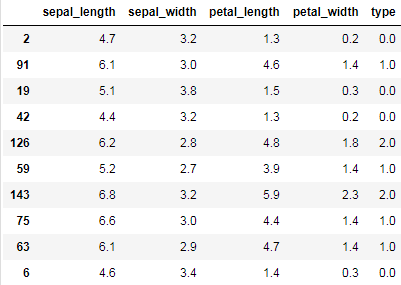注意：column_stack会先将一维数组转换为2维列向量后，按列水平进行拼接

numpy拼接小知识补充

np.column_stack([iris.data, iris.target])


等价于：

np.hstack([iris.data, iris.target.reshape(-1, 1)])


或：

np.concatenate([iris.data, iris.target.reshape(-1, 1)], axis=1)


或：

np.c_[iris.data, iris.target.reshape(-1, 1)]


iris.target.reshape(-1, 1)也可以用新增轴来表示，等价于：

iris.target[:, np.newaxis]


np.newaxis的本质等于None，可以直接用None替换，即：

iris.target[:, None]


个人觉得column_stack最方便，因为实现了将一维数组自动转换为2维列向量。

下面计算鸢尾花数据中，每个类别出现的频数

frequency = data["type"].value_counts()
frequency

2.0    50
1.0    50
0.0    50
Name: type, dtype: int64


将频数除以总数即表示每个类别出现的频率，使用百分比表示：

percentage = frequency * 100 / len(data)
percentage

2.0    33.333333
1.0    33.333333
0.0    33.333333
Name: type, dtype: float64


## 反映趋中趋势的几个指标

有均值、中位数、众数和分位数。

### 均值、中位数和众数

均值，即平均值，其为—组数据的总和除以数据的个数。

中位数，将一组数据升序排列，位于该组数据最中间位置的值，就是中位数。如果数据个数为偶数，则取中间两个数值的均值。

众数，一组数据中出现次数最多的值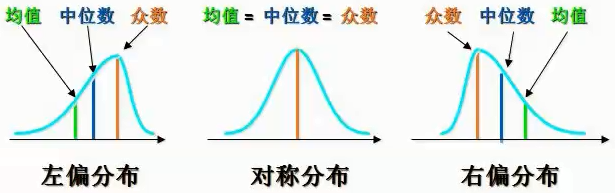从上图可以看到在正态分布下，三者是相同的，在偏态分布下，三者会有所不同。

数值变量在正态分布时，可以使用均值与中值表示集中趋势。在偏态分布下，均值容易受极端值的影响，所以一般使用中值表示集中趋势。

类别变量通常使用众数表示集中趋势，但众数在一组数据中可能不是唯一的。

举个例子，要统计居民的总体收入水平，使用哪项指标衡量更合适呢？首先收入属于数值变量，可以使用均值与中位数表示集中趋势。

但20%的人掌握着80%的人财富，居民收入是个严重右偏的分布，均值会受极端值的影响，所以使用中位数指标更合适。

下面我们计算花萼长度的均值，中位数以及众数：

# 计算花萼长度的均值。
mean = data["sepal_length"].mean()
# 计算花萼长度的中位数。
median = data["sepal_length"].median()
# 计算花萼长度的众数。
mode = data["sepal_length"].mode()
print(mean, median)
# mode方法返回的是Series类型。
print(mode)

5.843333333333335 5.8
0    5.0
dtype: float64


也可以使用scipy的stats模块来求一组数据的众数。

from scipy import stats
stats.mode(data["sepal_length"])

ModeResult(mode=array([5.]), count=array())


同时会返回该众数出现的频次。

看看分布：

# 绘制数据的分布（直方图 + 密度图）。
sns.distplot(data["sepal_length"])
# 绘制垂直线。
plt.axvline(mean, ls="-", color="r", label="均值")
plt.axvline(median, ls="-", color="g", label="中值")
plt.axvline(mode, ls="-", color="indigo", label="众数")
plt.legend()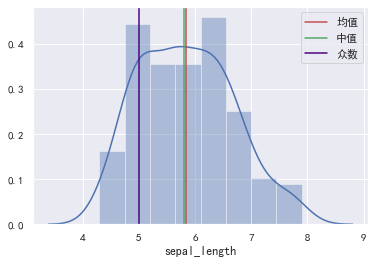Serise的mode方法和stats.mode()方法的区别

Serise的mode方法的返回值类型是Serise。stats.mode()方法的返回值类型是 ModeResult

如果众数的值不唯一，Series的mode()方法会显示所有众数，而stats.mode()方法只显示其中一个，但同时能知道该众数的个数。

s = pd.Series([1, 1, 1, 2, 2, 2, 3, 3, 3, 4, 5])


对于Series的mode方法：

s.mode()

0    1
1    2
2    3
dtype: int64


对于stats.mode()方法：

stats.mode(s)

ModeResult(mode=array(, dtype=int64), count=array())


### 分位数

分位数，通过n-1个分位将数据划分为n个区间，使得每个区间的数值个数相等（或近似相等）。其中，n为分位数的数量．常用的分位数有四分位数与百分位数。

以四分位数为例，通过3个分位，将数据划分为4个区间（百分位数可根据四分位数对比理解）。

• 第1个分位称为1/4分位（下四分位）数据中1/4的数据小于该分位值。
• 第2个分位称为2/4分位（中四分位）数据中2/4的数据小于该分位值。
• 第3个分位称为3/4分位（上四分位）数据中3/4的数据小于该分位值。

使用Numpy中计算分位数：

x = [1, 3, 10, 15, 18, 20, 23, 40]
# quantile与percentile都可以计算分位数，不同的是，quantile方法，
# q（要计算的分位数）的取值范围为[0, 1]，而percentile方法，q的取值范围为[0, 100]。
print(np.quantile(x, q=[0.25, 0.5, 0.75]))
print(np.percentile(x, q=[25, 50, 75]))

[ 8.25 16.5  20.75]
[ 8.25 16.5  20.75]


使用Pandas中计算分位数：

x = [1, 3, 10, 15, 18, 20, 23, 40]
s = pd.Series(x)
s.describe()

count     8.000000
mean     16.250000
std      12.395276
min       1.000000
25%       8.250000
50%      16.500000
75%      20.750000
max      40.000000
dtype: float64


describe方法支持自定义分位位置：

s.describe(percentiles=[0.25, 0.9])

count     9.000000
mean     16.777778
std      11.702326
min       1.000000
25%      10.000000
50%      18.000000
90%      26.400000
max      40.000000
dtype: float64


分位数计算的原理

首先计算分位点所在的索引位置：

x = np.array([1, 3, 10, 15, 18, 20, 23, 40])
n = len(x)
# 计算四分位的索引（index）。
q1_index = (n - 1) * 0.25
q2_index = (n - 1) * 0.5
q3_index = (n - 1) * 0.75
print(q1_index, q2_index, q3_index)

1.75 3.5 5.25


索引位置不是整数时，使用最近位置的两个整数，加权计算来得到四分位的位置，每个整数的权重为距离的反比。加权计算：

index = np.array([q1_index, q2_index, q3_index])
# 计算左边元素的值。
left = np.floor(index).astype(np.int8)
# 计算右边元素的值。
right = np.ceil(index).astype(np.int8)
# 获取index的小数部分与整数部分。
weight, _ = np.modf(index)
# 根据左右两边的整数，加权计算四分位数的值。权重与距离成反比。
q = x[left] * (1 - weight) + x[right] * weight
print(q)

[ 8.25 16.5  20.75]


当索引位置是整数时，计算过程可以简化为：

x = np.array([1, 3, 10, 15, 18, 20, 21, 23, 40])
n = len(x)
# 计算四分位的索引（index）。
index = (np.array([0.25, 0.5, 0.75])*(n - 1)).astype(np.int8)
print(x[index])

[10, 18, 21]


## 反映离散程度的极差、方差和标准差

极差指一组数据中，最大值与最小值之差。

方差体现的是一组数据中，每个元素与均值偏离的大小。

σ 2 = 1 n − 1 ∑ i = 1 n ( x i − x ˉ ) 2 \Huge{\sigma^{2}=\frac{1}{n-1} \sum_{i=1}^{n}\left(x_{i}-\bar{x}\right)^{2}}

• x i x_i ：数组中的每个元素。
• n：数组元素的个数。
• x ˉ \bar{x} ：数组中所有元素的均值。

标准差为方差的开方。

σ = 1 n − 1 ∑ i = 1 n ( x i − x ˉ ) 2 \Huge{\sigma=\sqrt{\frac{1}{n-1} \sum_{i=1}^{n}\left(x_{i}-\bar{x}\right)^{2}}}

关于三者，说明如下：

• 极差的计算非常简单，但是极差没有充分的利用数据信息。
• 方差（标准差）可以体现数据的分散性，方差（标准差）越大，数据越分散，方差（标准差）越小，数据越集中。
• 方差（标准差）也可以体现数娼的波动性（稳定性）。方差（标准差）越大，数据波动性越大，方差（标淮差）越小，数据波动性越小。
• 当数据较大时，也可以使用n代替n-1
# 计算极差。
sub = data["sepal_length"].max() - data["sepal_length"].min()
# 计算方差。
var = data["sepal_length"].var()
# 计算标准差。
std = data["sepal_length"].std()
print(sub, var, std)

3.6000000000000005 0.6856935123042505 0.8280661279778629


花瓣长度的方差较大，花瓣宽度的方差较小，绘图对比：

plt.figure(figsize=(15, 4))
plt.ylim(-0.5, 1.5)
plt.plot(data["petal_length"], np.zeros(len(data)), ls="",
marker="o", ms=10, color="g", label="花瓣长度")
plt.plot(data["petal_width"], np.ones(len(data)), ls="",
marker="o", ms=10, color="r", label="花瓣宽度")
plt.axvline(data["petal_length"].mean(), ls="--", color="g", label="花瓣长度均值")
plt.axvline(data["petal_width"].mean(), ls="--", color="r", label="花瓣宽度均值")
plt.legend()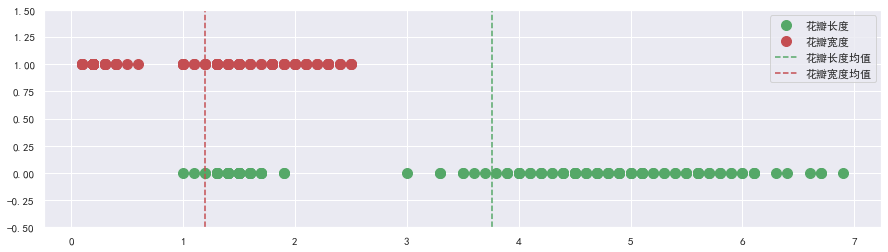## 反映分布形状的偏度和峰度

### 偏度

偏度是统计数据分布偏斜方向和程度的度量，是统计数据分布非对称程度的数字特征。

• 如果数据对称分布（例如正态分布），则偏度为0。
• 如果数据左偏分布．则偏度小于O。
• 如果数据右偏分布．则偏度大于0。
# 构造左偏分布数据。
t1 = np.random.randint(1, 11, size=100)
t2 = np.random.randint(11, 21, size=500)
t3 = np.concatenate([t1, t2])
left_skew = pd.Series(t3)
# 构造右偏分布数据。
t1 = np.random.randint(1, 11, size=500)
t2 = np.random.randint(11, 21, size=100)
t3 = np.concatenate([t1, t2])
right_skew = pd.Series(t3)
# 计算偏度。
print(left_skew.skew(), right_skew.skew())
# 绘制核密度图。
plt.legend()

-0.9911238058650503 0.7820903371872946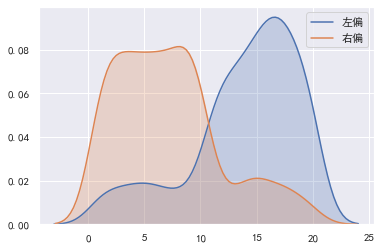### 峰度

峰度是描述总体中所有取值分布形态陡缓程度的统计量。可以将峰度理解为数据分布的高矮程度。峰度的比较是相对于标准正态分布的。

• 对于标准正态分布，峰度为0．
• 如果峰度大于0，则密度图高于标准正态分布。
• 数据在分布上比标准正态分布密集，方差（标淮羞）较小．
• 如果峰度小于0，则密度图低于标准正态分布。
• 说明数据在分布上比标准正态分布分散，方差（标准差）较大。
# 标准正态分布。
standard_normal = pd.Series(np.random.normal(0, 1, size=10000))
print("标准正态分布峰度：", standard_normal.kurt(), "标准差：", standard_normal.std())
print("花萼宽度峰度：", data["sepal_width"].kurt(), "标准差：", data["sepal_width"].std())
print("花瓣长度峰度：", data["petal_length"].kurt(), "标准差：", data["petal_length"].std())
sns.kdeplot(standard_normal, label="标准正态分布")
sns.kdeplot(data["sepal_width"], label="花萼宽度")
sns.kdeplot(data["petal_length"], label="花瓣长度")

标准正态分布峰度： 0.02338847301358893 标准差： 0.9980947521404823
花萼宽度峰度： 0.2907810623654279 标准差： 0.4335943113621737
花瓣长度峰度： -1.4019208006454036 标准差： 1.7644204199522617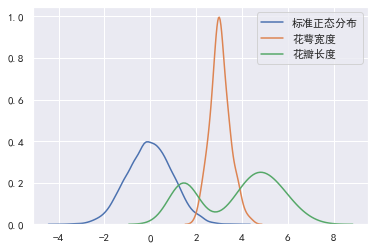## 绘制对数正态分布的图像

如果一个分布取对数后为正态分布，则该分布称为对数正态分布。

import random
import numpy as np

logdata = [random.lognormvariate(0, 0.6) for i in range(100000)]
data = np.random.normal(0, 0.6, size=100000)
sns.kdeplot(data, label="正态分布")
sns.kdeplot(np.log(logdata), label="对数正态分布取对数")
sns.kdeplot(np.exp(data), label="正态分布取幂")
sns.kdeplot(logdata, label="对数正态分布")
plt.xlim(-5, 5)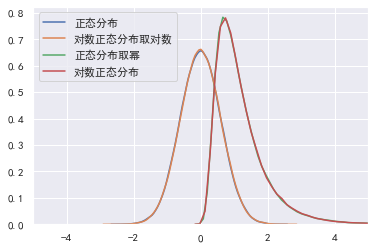# 推断统计分析

## 推断统计的概念

总体，就是被研究的全部数据，总体中的某个数据，就是个体。从总体中抽取部分个体．就构成了样本，样本中包含的个体数量，称为样本容量

在实际的研究中，往往无法获取全部数据，只能对总体进行抽样。推断统计就是研究根据样本数据去推断总体数量特征的方法，它在对样本数据进行描述的基础上，对统计总体的未知数量特征做出以概率形式表述的推断，从而通过样本统计量来估计总体参数。

推断统计分析分为参数估计和假设检验。

## 参数估计

### 点估计与区间估计

点估计，就是使用样本的统计量去代替总体参数。例如，我们要求鸢尾花的平均花瓣长度，就可以使用样本的均值来估计总体的均值：

import numpy as np
import pandas as pd
import matplotlib.pyplot as plt
import seaborn as sns
from sklearn.datasets import load_iris

# 设置seaborn绘图的样式。
sns.set(style="darkgrid")
plt.rcParams["font.family"] = "SimHei"
plt.rcParams["axes.unicode_minus"] = False

iris = load_iris()
data = np.column_stack((iris.data, iris.target))
data = pd.DataFrame(data,
columns=["sepal_length", "sepal_width", "petal_length", "petal_width", "type"])
print(data["petal_length"].mean())

3.7586666666666693


点估计实现简单，但是容易受到随机抽样的影响，无法保证结论的准确性。

区间估计则根据样本的统计量，计算出一个可能的区间与概率，表示总体的参数会有多少概率位于该区间中。区间估计指定的区间，我们称为置信区间，而区间估计指定的概率，称为置信度。例如，鸢尾花花瓣长度有70%的可能性在3.4cm-3.8cm之间，3.4cm- 3.8cm就是置信区间，而70%就是置信度。

总之，点估计是使用一个值来代替总体的参数值，能够给出具体的估计值但缺乏准确性。区间估计是使用的一个置信区间与置信度，表示总体参数有多少可能（置信度）会在该范围（置信区间）内，能够给出合理的范围和支持概率。

经过抽样，获取—个样本之后，该如何才能确定置信区间与置信度呢？区间估计的基石就是根据中心极限定理。

### 中心极限定理

定理内容：如果总体（分布不重要）均值为μ，方差为 σ 2 \sigma^{2} 。我们进行随机抽样，样本容量为n，当n增大时，则样本均值逐渐趋近服从正态分布： X ˉ ∼ N ( μ , σ 2 / n ) \bar{X}\sim N\left(\mu, \sigma^{2} / n\right)

该定理说明了总体与样本之间，在分布上的联系。该定理说明在抽样的样本容量n足够大时，进行多次抽样．则每次抽样会得到—个均值，这些均值会围绕在总体均值左右，呈正态分布。均值等于总体的均值，标准差等于总体标准差 σ \sigma 除以 n \sqrt{n}

程序模拟

下面模拟总体的均值为30，标准差为80，抽样的样本容量n为64，看看1000次抽样的样本均值是否构成均值为30，标准差为10的正态分布：

data = np.random.normal(30, 80, 100000)
mean_arr = np.zeros(1000)
for i in range(1000):
mean_arr[i] = np.random.choice(data, size=64, replace=False).mean()
print("均值：", np.mean(mean_arr))
print("标准差：", np.std(mean_arr), 80/np.sqrt(64))
print("偏度：", pd.Series(mean_arr).skew())
print("峰度：", pd.Series(mean_arr).kurt())
sns.distplot(mean_arr)

均值： 29.98515540795136
标准差： 10.387123594380887 10.0
偏度： -0.0499634202251343
峰度： 0.1875444656831493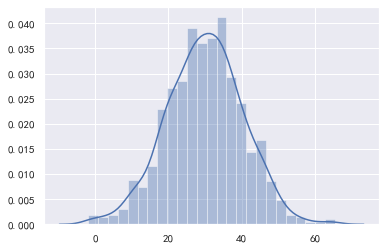从上述结果可以看到，样本的均值和标准差接近于理论值。

### 正态分布的特性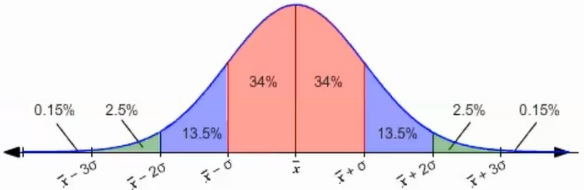在正态分布中，数据的分布比例如下：

• 以均值为中心，在1倍标准差内 ( x ˉ − σ , x ˉ + σ ) (\bar{x}-\sigma, \bar{x}+\sigma) ，包含约68%的样本数据。
• 以均值为中心，在2倍标准差内 ( x ˉ − 2 σ , x ˉ + 2 σ ) (\bar{x}-2\sigma, \bar{x}+2\sigma) ，包含约95%的样本数据。
• 以均值为中心，在3倍标准差内 ( x ˉ − 3 σ , x ˉ + 3 σ ) (\bar{x}-3\sigma, \bar{x}+3\sigma) ，包含约99.7%的样本数据。

可以用程序模拟一下：

scale = 50
x = np.random.normal(0, scale, size=10000000)
for times in np.arange(1, 4):
y = x[(x > -times * scale) & (x < times * scale)]
print(f"{times}倍标准差：{len(y)/len(x):.1%}")

1倍标准差：68.3%
2倍标准差：95.4%
3倍标准差：99.7%


二分搜索查找当正态分布覆盖99%样本数据时，大概是多少倍标准差：

import numpy as np
scale = 1
x = np.random.normal(0, scale, size=100000000)
confidenceLevel = 99
arr = np.arange(2, 3, 0.00001)
iterations = 0

low, high = 0, len(arr) - 1
while low <= high:
mid = (high + low) >> 1
times = arr[mid]
rate = len(x[(x > -times * scale) & (x < times * scale)]) * 100 / len(x)
if rate >= confidenceLevel:
high = mid - 1
else:
low = mid + 1
iterations += 1
result = round(arr[low], 4)
print(result, "迭代次数：", iterations)

2.5761 迭代次数： 17


可以看到标准差大概是2.58倍时，正态分布覆盖99%样本数据。

可以通过scipy获取准确值：

from scipy.stats import norm

def calcTimes(confidenceLevel):
alpha = 1 - confidenceLevel
return norm.ppf(1 - alpha / 2)

calcTimes(0.99)


结果：

2.5758293035489004


执行calcTimes(0.95)的结果是1.959963984540054，说明1.96倍标准差能覆盖95%的样本数据。

计算在正态分布情况下，指定倍数的标准差能覆盖多大比例的样本数据可以使用以下命令：

from scipy.stats import norm
# norm.cdf(x=?)计算正态分布概率（面积）：P(X<x)

def calcArea(times):
return (norm.cdf(x=times) - norm.cdf(x=-times))*100

calcArea(2.58)


结果：

99.01199684844586


### 置信度与置信区间

根据中心极限定理，如果多次抽样（总体均值 μ \mu ，方差为 σ 2 \sigma^2 ）,则样本均值( x ˉ \bar{x} )构成正态分布，满足 X ˉ ∼ N ( μ , σ 2 / n ) \bar{X}\sim N\left(\mu, \sigma^{2}/n\right) 。如果我们对总体进行一次抽样，则该样本的均值有95%的概率会在 ( μ − 2 σ , μ + 2 σ ) (\mu-2\sigma, \mu+2\sigma) ，仅有5%的概率会在 ( μ − 2 σ , μ + 2 σ ) (\mu-2\sigma, \mu+2\sigma) 范围外。根据小概率事件（很小的概率在一次抽样中基本不会发生），如果抽样的样本均值在 ( μ − 2 σ , μ + 2 σ ) (\mu-2\sigma, \mu+2\sigma) 之外，我们就可以认为，本次抽样来自总体的均值并非我们所期望的均值。

通常，我们以2倍标准差作为判断依据，则以样本均值为中心，正负2倍标准差构成的区间，就是置信区间。而2倍标准差包含了95%的数据，因此此时置信度为95%。即我们有95%的信心认为，总体均值会在置信区间之内。

下面，我们模拟进行一次抽样，看看实际的总体均值是否会在置信区间之内呢：

mean = np.random.randint(-10000, 10000)  #总体均值
std = 50  # 定义总体标准差
n = 50  # 样本容量
data = np.random.normal(loc=mean, scale=std, size=10000)
sample = np.random.choice(data, size=n, replace=False)
sample_mean = sample.mean()
print("总体均值：", mean)
print("一次抽样的样本均值：", sample_mean)
plt.plot(mean, 0, marker="*", color="orange", ms=15, label="总体均值")
plt.plot(sample_mean, 0, marker="o", color="r", label="样本均值")

se = std / np.sqrt(n)
min_ = sample_mean - 1.96 * se
max_ = sample_mean + 1.96 * se
print("置信区间（95%置信度）", (min_, max_))
plt.hlines(0, xmin=min_, xmax=max_, colors="b", label="置信区间")
plt.axvline(min_, ymin=0.4, ymax=0.6, color="r", ls="--", label="左边界")
plt.axvline(max_, ymin=0.4, ymax=0.6, color="g", ls="--", label="右边界")
plt.legend()

总体均值： 8819
一次抽样的样本均值： 8824.987475450054
置信区间（95%置信度） (8811.128182538798, 8838.84676836131)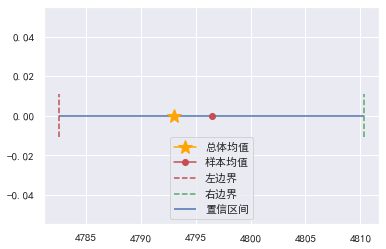还可以直接通过scipy计算：

from scipy import stats
stats.norm.interval(0.95, loc=sample.mean(), scale=std / np.sqrt(n))


结果同样为(8811.128437206558, 8838.84651369355)

实例

经过大量长期统计，A公司商品日均生产量为50，标准差为15。公司领导对最近半个月（15天）的生产量进行突击检查，请问：

• 如果最近半个月日均产量为45．是否可能存在消极变数?例如，机器老化．员工怠工等。
• 如果最近半个月日均产量为59，是否可能存在积极改进?例如，提高生产效率，加大人力投入等。
mean, std = 50, 15
n = 15
sigma = std / np.sqrt(n)
start, end = stats.norm.interval(0.95, loc=mean, scale=sigma)
print(f"样本均值有95%的概率在{start:.1f}-{end:.1f}范围内")

样本均值有95%的概率在42.4-57.591范围内


因此日均产量为45在合理范围内，日均产量为59很可能存在积极改进。

### 总体标准差未知时的区间估计

上述区间估计的方法，适用于已知总体标准差且样本量大于30的情况下。但正常情况下，我们对总体的标准差都是未知的，有时抽样样本量也会少于30。

不过，进行多次抽样，样本的均值服从t分布，当每次抽样样本量n增大时（达到30以上），t分布将逐渐接近于正态分布。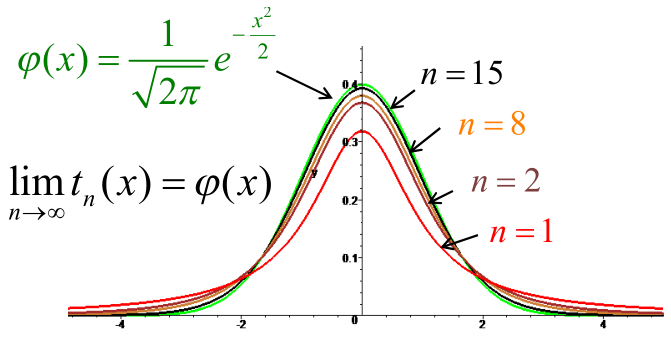使用 t 分布的好处是不需要知道总体的标准差，可以直接根据样本的标准差进行计算。

于是我们可以根据鸢尾花花瓣长度的样本数据，去估算在95%置信度下全球鸢尾花花瓣长度的均值。

首先导包并加载数据：

import pandas as pd
from sklearn.datasets import load_iris
from scipy import stats

data = pd.DataFrame(
iris.data,
columns=["sepal_length", "sepal_width", "petal_length", "petal_width"])


计算：

confidenceLevel = 0.95
mean = data.petal_length.mean()
start, end = stats.t.interval(alpha=confidenceLevel, df=len(data) - 1, loc=mean, scale=stats.sem(data.petal_length))
print(f"样本均值是{mean:.2f}，在{confidenceLevel:.0%}置信度下，全球鸢尾花花瓣长度的均值在{start:.2f}-{end:.2f}cm的范围")


结果：样本均值是3.76，在95%置信度下，全球鸢尾花花瓣长度的均值在3.47-4.04cm的范围

## 假设检验

示例

某车间用一台包装机包装葡萄糖，袋装糖的净重是—个随机变量．它服从正态分布。当机器正常时，其均值为0.5kg，标准差为0.015kg。某日开工后为检验包装机是否正常，随机地抽取它所包装的糖9袋，称得净重为(kg)

0.497, 0.506, 0.518, 0.524, 0.498, 0.511, 0.520, 0.515, 0.512

：机器是否正常？

按照前面区间估计的做法，我们可以根据总体的特征计算出样本均值的置信区间，然后判断当前样本的均值是否存在于这个范围之内：

import numpy as np
from scipy import stats

# 总体的均值和标准差
mean, std = 0.5, 0.015
# 样本
a = np.array([0.497, 0.506, 0.518, 0.524, 0.498, 0.511, 0.520, 0.515, 0.512])
left, right = stats.norm.interval(0.95, loc=mean, scale=std / np.sqrt(len(a)))
print(f"95%置信度下，样本均值的置信区间：({left:0.3f},{right:0.3f})")
print("样本均值：", a.mean())


结果：

95%置信度下，样本均值的置信区间：(0.490,0.510)
样本均值： 0.5112222222222224


显然样本均值不在根据总体估算的置信区间之内，所以在95%置信度下，机器是不正常的。

另一种方案就是根据样本去估计总体，由于样本量为9小于30，所以我们使用t分布来估计总体的均值：

import numpy as np
from scipy import stats

# 总体的均值和标准差
mean, std = 0.5, 0.015
# 样本
a = np.array([0.497, 0.506, 0.518, 0.524, 0.498, 0.511, 0.520, 0.515, 0.512])
left, right = stats.t.interval(0.95, df=len(a) - 1, loc=a.mean(), scale=stats.sem(a))
print(f"95%置信度下，总体均值的置信区间：({left:0.3f},{right:0.3f})")
print("总体实际均值：", mean)


结果：

95%置信度下，总体均值的置信区间：(0.504,0.518)
总体实际均值： 0.5


显然95%置信度下总体实际均值也不在总体均值的置信区间之内，所以我们可以认为机器是不正常的。

### 假设检验的概念

假设检验的思路下，则是先给出一个原假设，然后根据反证法看是否出现小概率事件，如果没有出现小概率事件的情况下则接受原假设，若出现小概率事件情况下则拒绝原假设接受备择假设。

对于上面这个问题的思路就是，先作原假设：机器是正常的，备择假设：机器是不正常的。结果样本均值不在置信区间内，出现了小概率事件，所以拒绝原假设接受备择假设：机器是不正常的。

假设检验，也称为显著性检验，是通过样本的统计量，来判断与总体参数之间的差异是否显著。原假设也称为零假设或 H 0 H_0 ，备则假设也称为对立假设或 H 1 H_1

根据样本信息进行分析判断，是选择接受（维持）原假设还是拒绝原假设（接受备择假设）。

在假设检验中，我们认为，小概率事件在—次试验中是不会发生的。—旦小慨率事件发生，则我们就有理由拒绝原假设。

假设检验遵循"疑罪从无"的原则，接受原假设，并不代表原假设—定是正确的，只是没有充分的证据，去证明原假设是错误的，因此，只能维持原假设。

### P-Value与显著性水平

P-Value是一个概率值，表示支持原假设的概率。在原假设为等值假设时，P-Value也表示样本统计量与总体参数无差异的概率。

显著性水平则是我们预先设定的—个阈值，使用 α \alpha 表示，通常 α \alpha 的取值为0.05（1-a为置信度）。当P-Value的值大于 α \alpha 时，支持原假设，否则，拒绝原假设。

假设检验的步骤如下:

1. 设置原假设与备择假设。
2. 设置显著性水平 α \alpha （通常选择 α \alpha = 0.05）。
3. 根据问题选择假设检验的方式。
4. 计算统计量，并通过统计量获取 P P 值。
5. 根据 P P 值与 α \alpha 值，决定接受原假设还是备择假设。

### Z检验与t检验

Z检验用来判断样本均值是否与总体均值具有显著性差异。Z检验要求总体呈正态分布、总体方差已知，否则无法使用Z检验，另外要求样本容量最好大于30，否则建议使用t检验。

Z统计量的计算方式如下：

Z = x ˉ − μ 0 S x ˉ = x ˉ − μ 0 σ / n \Huge{Z=\frac{\bar{x}-\mu_{0}}{S_{\bar{x}}}=\frac{\bar{x}-\mu_{0}}{\sigma / \sqrt{n}}}

• x ˉ \bar{x} ：样本均值。
• μ 0 \mu_{0} ：待检验的总体均值（假设的总体均值）。
• S x ˉ S_{\bar{x}} ：样本均值分布的标准差（标准误差）。
• σ \sigma ：总体的标准差。
• n n ：样本容量。

通过假设检验来求解前面的示例：

1. 设置原假设与备择假设。
• 原假设： μ = μ 0 = 0.5 k g \mu=\mu_0 = 0.5kg （机器运作正常）
• 备择假设： μ ≠ μ 0 ≠ 0.5 k g \mu \neq \mu_0 \neq 0.5 kg （机器运作不正常）
2. 设置显著性水平。
• 设置 α \alpha = 0.05
3. 根据问题选择假设检验的方式。
• 袋装糖的净重呈正态分布，总体标准差已知，选择 Z Z 检验。
4. 计算统计量，并通过统计量获取 P P 值。
• 根据选择的假设检验，进行计算。
import numpy as np
from scipy import stats

# 总体的均值和标准差
mean, std = 0.5, 0.015
# 样本
a = np.array([0.497, 0.506, 0.518, 0.524, 0.498, 0.511, 0.520, 0.515, 0.512])
# 样本均值
sample_mean = a.mean()
sigma = std / np.sqrt(len(a))

Z = (sample_mean - mean) / sigma
print("统计量Z:", Z)
P = 2 * stats.norm.sf(abs(Z))
print("P-Value值：", P)


结果：

统计量Z: 2.244444444444471
P-Value值： 0.02480381963225589

1. 根据P值与α值，决定接受原假设还是备择假设。

• 由结果可知， P P 值即支持原假设的概率小于显著性水平α的值0.05，故我们拒绝原假设。接受备择假设，即我们认为机器运作是不正常的。

这就是假设检验的步骤。

t检验则适用于总体呈正态分布且方差未知的情况。

随着样本容量的增大（样本量达到30以上时），t分布逐渐接近于正态分布。此时，t检验也就近似应用于Z检验。

t统计量的计算方式如下：

t = x ˉ − μ 0 S x ˉ = x ˉ − μ 0 S / n \Huge{t=\frac{\bar{x}-\mu_{0}}{S_{\bar{x}}}=\frac{\bar{x}-\mu_{0}}{S / \sqrt{n}}}

• x ˉ \bar{x} ：样本均值。
• μ 0 \mu_{0} ：待检验的总体均值（假设的总体均值）。
• S x ˉ S_{\bar{x}} ：样本均值的标准差（标准误差）。
• S S ：样本的标准差。
• n n ：样本容量。

鸢尾花花瓣长度的均值为3.5cm，根据假设检验的步骤判断是否正确：

1. 设置原假设与备择假设。
• 原假设：总体均值 μ = μ 0 = 3.5 c m \mu=\mu_0 = 3.5cm （该说法正确）
• 备择假设：总体均值 μ = μ 0 ≠ 3.5 c m \mu = \mu_0 \neq 3.5cm （该说法不正确）
2. 设置显著性水平。
• 设置 α \alpha = 0.05
3. 根据问题选择假设检验的方式。
• 鸢尾花呈正态分布，总体标准差未知，选择 t t 检验。
4. 计算统计量，并通过统计量获取 P P 值。
import pandas as pd
from sklearn.datasets import load_iris
import numpy as np

data = pd.DataFrame(
iris.data,
columns=["sepal_length", "sepal_width", "petal_length", "petal_width"])
mean = data["petal_length"].mean()
std = data["petal_length"].std()
print("样本均值：", mean, "样本标准差:", std)

t = (mean - 3.5) / (std / np.sqrt(len(data)))
print("t统计量:", t)

# 计算p值
# df:自由度，即变量可以自由取值的个数
P = 2 * stats.t.sf(abs(t), df=len(data) - 1)
print("P-Value值：", P)

# 还可以通过scipy提供的相关方法来进行t检验的计算，无需自行计算。
t, p_twoTail = stats.ttest_1samp(data['petal_length'], 3.5)
print(f"t统计量:{t}, P-Value值：{p_twoTail}")

样本均值： 3.7586666666666693 样本标准差: 1.7644204199522617
t统计量: 1.7954942587239626
P-Value值： 0.07460161706985045


还可以直接通过scipy提供的相关方法来进行t检验的计算：

t, p_twoTail = stats.ttest_1samp(data['petal_length'], 3.5)
print(f"t统计量:{t}, P-Value值：{p_twoTail}")


结果：

t统计量:1.79549425872394, P-Value值：0.07460161706985409


P P 值即支持原假设的概率大于显著性水平α的值0.05，故我们没有充足的理由拒绝原假设，则接受原假设，即我们认为鸢尾花花瓣长度的均值为3.5cm。

### 双边检验与单边检验

• 原假设： μ = μ 0 = 3.5 c m \mu=\mu_0 = 3.5cm
• 备择假设： μ ≠ μ 0 ≠ 3.5 c m \mu \neq \mu_0 \neq 3.5cm

对于上面的等值假设，检验的是总体均值( μ \mu )与假设均值( μ 0 \mu_0 )是否相等，当 μ ≠ μ 0 \mu≠\mu_0 时．总体均值可以大于假设均值，也可以小于假设均值，像这样的检验称为双边假设检验（双边检验）。非等值假设时，总体参数大于或者小于假设参数值．像这样的检验称为单边假设检验(单边检验)。例如，我们仅关注技术改进后是否比以前有所提高，而不是关注是否与以前不同。

以均值为例，设总体均值为 μ \mu ，假设均值为 μ 0 \mu_0

如果设立：

• 原假设: μ ≤ μ 0 \mu \leq \mu_{0}
• 备择假设: μ > μ 0 \mu > \mu_{0}
则称这样的假设为右边假设检验(右边检验)

如果设立：

• 原假设: μ ≥ μ 0 \mu \geq \mu_{0}
• 备择假设: μ < μ 0 \mu < \mu_{0}
则称这样的假设为左边假设检验(左边检验)

说明：在单边检验中，原假设为维持现状，备则假设为改变现状。

我们可以根据计算P值的方式来记忆是左边假设检验还是右边假设检验，

例如 μ ≤ μ 0 \mu \leq \mu_{0} 等价于 μ − μ 0 ≤ 0 \mu - \mu_{0} \leq 0 ，那么应该使统计量越小P值越大，就应该计算统计量右边围成的面积。

μ ≥ μ 0 \mu \geq \mu_{0} 等价于 μ − μ 0 ≥ 0 \mu - \mu_{0} \geq 0 则应该计算左边围成的面积。

所以对于单边检验，左边和右边是指应该计算统计量左边还是右边围成的面积。

而对于等值假设，即双边检验，应该使统计量越接近0，P值越大，应该计算统计量对应左右两侧位置与两边围成的面积。

例如，判断鸢尾花的平均花瓣长度等于3.5cm的说法是否正确，计算出统计量为1.8后，再计算左右两边围成的面积即为P值：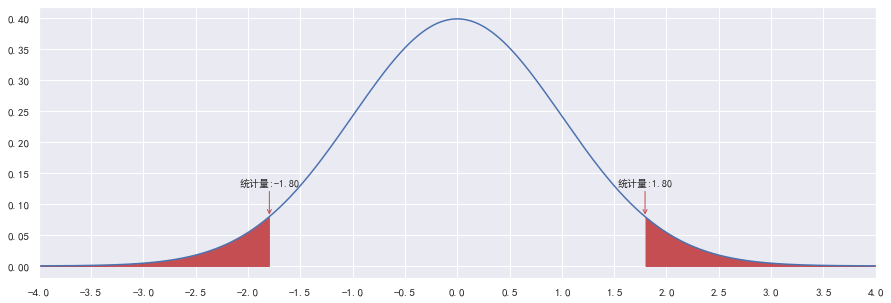### 右边假设检验

判断鸢尾花的平均花瓣长度不超过3.5cm的说法是否正确

1. 设置原假设与备择假设。
• 原假设： μ ≤ μ 0 \mu \leq \mu_0
• 备择假设： μ > μ 0 \mu > \mu_0
2. 设置显著性水平。
• 设置 α \alpha = 0.05
3. 根据问题选择假设检验的方式。
• 鸢尾花呈正态分布，总体标准差未知，选择 t t 检验。
4. 计算统计量，并通过统计量获取 P P 值。

计算下方红色部分（右边）的面积：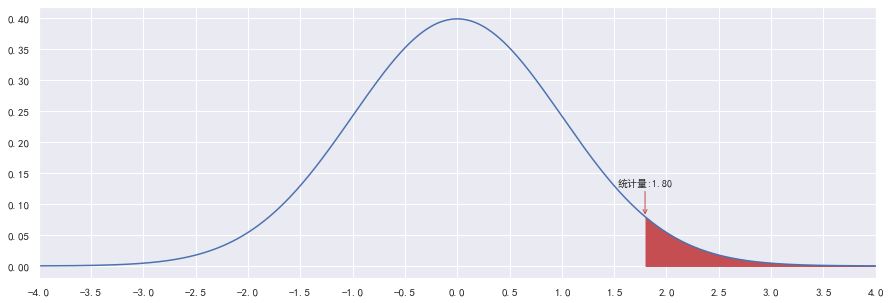print("t统计量:", t)
P = stats.t.sf(t, df=len(data) - 1)
print("P-Value值：", P)

t统计量: 1.79549425872394
P-Value值： 0.037300808534927045

1. 根据P值与α值，决定接受原假设还是备择假设。

• P < α P<\alpha ，因此拒绝原假设，我们认为鸢尾花的平均花瓣长度超过3.5cm。

### 左边假设检验

假如判断鸢尾花的平均花瓣长度不小于3.5cm的说法是否正确

1. 设置原假设与备择假设。
• 原假设： μ ≥ μ 0 \mu \geq \mu_0
• 备择假设： μ < μ 0 \mu < \mu_0
2. 设置显著性水平。
• 设置 α \alpha = 0.05
3. 根据问题选择假设检验的方式。
• 鸢尾花呈正态分布，总体标准差未知，选择 t t 检验。
4. 计算统计量，并通过统计量获取 P P 值。

计算下方红色部分（左边）的面积：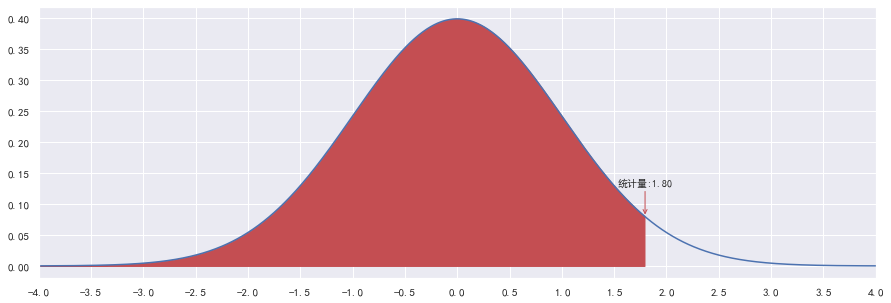print(t)
P = stats.t.cdf(t, df=len(data) - 1)
print("P-Value值:", P)

1.79549425872394
P-Value值: 0.962699191465073

1. 根据P值与𝛼值，决定接受原假设还是备择假设。

• P > α P>\alpha ，因此维持原假设，我们认为鸢尾花的平均花瓣确实长度不小于3.5cm

### 示例

某公司要求，平均日投诉量均值不得超过1%。现检查—个部门的服务情况。在该部门维护的—个500人客户群中，近7天的投诉量分别为5, 6, 8, 4, 4, 7, 0。请问该部门是否达标？

原假设平均日投诉量均值小于等于1%，是个右边假设检验，总体标准差未知，样本量小于30，选择 t t 检验：

data = np.array([5, 6, 8, 4, 4, 7, 0]) / 500 * 100
print(data)
# 假设 平均日投诉量 <=1%
mean = data.mean()
std = data.std()
print("样本均值：", mean, "样本标准差:", std)
t = (mean - 1) / (std / np.sqrt(len(data)))
print("t统计量:", t)
P = stats.t.sf(t, df=len(data) - 1)
print("P-Value值：", P)

[1.  1.2 1.6 0.8 0.8 1.4 0. ]
样本均值： 0.9714285714285715 样本标准差: 0.4831867007225075
t统计量: -0.1564465546936854
P-Value值： 0.5595938210714403


支持原假设的概率超过显著性水平，故接受原假设，即平均日投诉量均值没有超过1%，该部门达标。

展开全文• 1、错误描述2、错误原因 无标题文档 姓名：  用浏览器预览时，弹出提示窗口3、解决办法

1、错误描述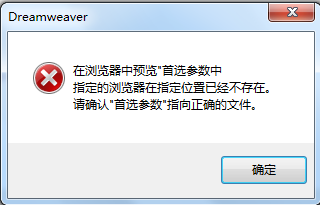2、错误原因

<!doctype html>
<html>
<meta charset="utf-8">
<title>无标题文档</title>
<script type="text/javascript" src="AngularSpec.js"></script>

<body>
<div ng-app="">
<p>姓名：<input type="text" ng-model="name"></p>
<p ng-bind="name"></p>
</div>
</body>
</html>


用浏览器预览时，弹出提示窗口；原因是原来用的浏览器已经卸载，再次用该浏览器预览，会导致出现错误

3、解决办法

（1）重新设置默认浏览器

（2）换其他浏览器预览

展开全文AngularJS
• 该楼层疑似违规已被系统折叠隐藏此楼查看此楼西工大机考《C语言程序设计》网考寻求答案(非免费)找我Q...，以下选项中对a数组元素正确引用的是( )。A.a[!1]B.aC.aD.a[1>2][!1]2. 以下描述错误的是...
• ## 关于S参数的一些理解

万次阅读 多人点赞 2017-10-17 20:55:52
本文将从S参数的定义，S参数的表达方式，S参数的特性，混合模式S参数，S参数测量等多个方面介绍S参数的一些最基本的知识。 1，S参数的定义 人们都喜欢用一句话来概括一个术语。 譬如用一句话S参数
• python开发工程师考证试题选择题题目关于 Python 程序格式框架的描述，以下选项中错误的是 ( A )A: Python 语言不采用严格的“缩进”来表明程序的格式框架B: Python 单层缩进代码属于之前最邻近的一行非缩进代码，...
• 今天我们在这里谈的tag标签的正确使用方法，就属于网站聚合页面优化的范凑。 tag标签优化是一种非常常见的网站优化手段。 百度百科的解释是：TAG标签是一种由您自己定义的，比分类更准确、更具体，可以概括文章...tag标签
• 参数的值是否改变与函数中对变量的操作有关，与参数类型无关答案∶D2、在Python中，关于全局变量和局部变量，以下选项中描述正确的是A.一个程序中的变量包含两类∶全局变量和局部变量B.全局变量...
• ## scala习题精选100道

万次阅读 2017-05-09 00:29:51
28、**[intermediate]**对于表达式 val tokens = "one two three four two two three four".split(" ") val map = new HashMap[String,Int] for(key ){ map(key) = map.getOrElse(key,0) + 1 }...scala
• ## yolo系列之yolo v3【深度解析】

万次阅读 多人点赞 2018-09-12 16:24:48
看过yolov3论文的应该都知道，这篇论文写得很随意，很多亮点都被作者都是草草描述。很多骚年入手yolo算法都是从v3才开始，这是不可能掌握yolo精髓的，因为v3很多东西是保留v2甚至v1的东西，而且v3的论文写得很随心。...
• ## Python 函数参数

千次阅读 多人点赞 2017-06-01 19:59:41
对于调用者来说，只需关注如何传递正确参数，以及函数的返回值就足够了，无需了解函数的内部实现（除非想学习、跟踪源码）。 话虽如此，但对于函数的定义来说，灵活性却非常大。除了常规定义的必选参数以外，还...Python Python函数 Python函数参数 Python可变参数 Python默认参数
• ## 【python技能树】python编码规范

千次阅读 多人点赞 2022-05-05 11:07:22
def FuncName(parameter1,parameter2): """ 描述函数要做的事情 :param parameter1: 参数描述(类型、用途等) :param parameter2: 参数描述 :return: 返回值描述 """ # We use a weighted dictionary search to ...python python技能树 python编码规范
• 8、 关于提示参数，以下描述正确的是？（ ABC ） A． 指定清晰、明确的提示信息， 准确地告知用户，需要提供什么样的信息。 B． 提示参数带有提示结束符（比如冒号或者问号） ，这样可以分开提示参数与用户输入，...python
• 【单选题】S 和 T 是两个集合,哪个选项对 S^T 的描述正确的?【单选题】关于 turtle 库的画笔控制函数,哪个选项的描述是错误的?【 】【单选题】哪个选项对 turtle.done() 的描述正确的?【 】【单选题】下列程序的...
• 1. MLE的意义：样本估计总体分布参数 假定一个事件的观测样本服从如下分布，我们如何确定总体数据的分布模型？ 首先应该想到是建立线性回归模型，然而由于该变量不是正态分布的，而且是不对称的，因此不符合线性...
• A概念模式B内模式C设计模式D外模式正确答案： D一个工作人员可使用多台计算机，而一台计算机被多个人使用，则实体工作人员与实体计算机之间的联系是___________。A多对一B多对多C一对一D一对多正确答案： B软件生命...
• 11、T是一个数据类型，关于std::vector::at 和 std::vector::operator[] 描述正确的是： at总是做边界检查， operator[] 不做边界检查. at 不做边界检查， operator[] 做边界检查. at和operator[] 是一样的...
• 1、关于Python对文件的处理，以下选项...可变参数在定义时只有两种变现形式: * param和 * param ,前者表示可以接受多个位置参数收集的实参组成一个元组 ,后者表示可以接收多个关键字参数收集的实参名和值组成一个字典。字符串 python 数据结构
• 然后，我们可以确定在哪些情况下Python方法是正确的，以及在哪些情况下可能滥用了该语言的特性。 1．如何将参数复制到函数中 Python中的第一条规则是所有参数都由一个值传递——总是这样。这意味着，当把值传递给...Python 编程语言 代码规范
• 提示：好多小伙伴反映，直接看到答案不...1、以下关于java封装的描述中，正确的是： 正确答案: A 你的答案: A (正确) 封装的主要作用在于对外隐藏内部实现细节，增强程序的安全性 封装的意义不大，因此在编码中尽量不要java
• 8、 关于提示参数，以下描述正确的是？（ ABC ） A． 指定清晰、明确的提示信息， 准确地告知用户，需要提供什么样的信息。 B． 提示参数带有提示结束符（比如冒号或者问号） ，这样可以分开提示参数与用户输入，看...
• ## USB描述符详细讲解

千次阅读 2018-04-09 10:39:31
在USB中USB HOST 是通过各种描述符来识别设备的，有 设备描述符，接口描述符，端点描述符，字符描述符，报告描述符等USB HID 设备是通过报告来传送数据的，报告有：输入报告 和 输出报告输入报告：是设备发送给主机...
• 1、IS-IS协议所使用的SNAP地址主要由哪几个部分构成？A.AREA ID B.DSCPC.SYSTEM ID ...2、下面关于OSPF报文描述正确的是？A.在ExStart状态下协商主从关系。确认主从关系之后，主路由器发送DD报文...
• 14、关于数据组织的维度描述正确的是 A.二维数据由对等关系的有序或无序数据构成 B.高维数据由关联关系数据构成 C.CSV是一维数据 D.一维数据采用线性方式存储 答案：D [解析]二维数据由关联关系的数据构成，因此A项...
• ## 推断统计：参数估计和假设检验

千次阅读 多人点赞 2020-03-03 00:35:24
目录 ...  3、参数估计(点估计和区间估计)    1）参数估计、点估计和区间统计的概念    2）点估计说明    3）区间估计说明   4、中心极限定理    1）中心极限定理的概念    2...
• ## JAVAEE选择题

千次阅读 2021-07-13 11:47:30
2、以下有关Bean的装配方式说法正确的是（C）。 C、在Spring实例化Bean的过程中，Spring首先会调用Bean的默认构造方法来实例化Bean对象，然后通过反射的方式调用setter方法来注入属性值； 注 :设值注入要求一个Bean...
• 18、下列关于makefile描述正确的是（）。 主要包含了五个东西：显式规则、隐晦规则、变量定义、文件指示和注释 makefile文件保存了编译器和连接器的参数选项 在Makefile不可以使用include关键字把别的...
• ## 2021HCIA-云计算题库（263题）

万次阅读 多人点赞 2021-04-20 15:03:14
1.在华为 FusionCompute 中，管理员可以查看虚拟机连接的 DVS 端口（） [单选题] * ...在描述中添加这是测试端口 3.在华为 FusionCompute 中，克隆虚拟机时不支持调整虚拟机的规格。（） [单选题] * A.TRUE B.FALSE云计算
• 1.保留主要的特征,同时减少下一层的参数和计算量，防止过拟合； 2. 保持某种不变性，包括translation(平移)，rotation(旋转)，scale(尺度),常用的有mean-pooling和max-pooling。 mean-pooling(平均值)  对一块小......